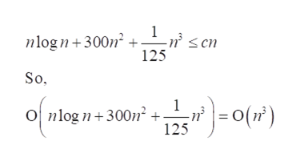# Order the following: O(n2), O(12 + 7n), O(n log(n) + 300 n2 + 1/125 n3)

Question
2 views
1. Order the following: O(n2), O(12 + 7n), O(n log(n) + 300 n2 + 1/125 n3)
check_circle

Step 1

The function is represented in the form of O(g(n)) if there is a positive integer( n0) and constant(c), such that,

Step 2

For O(12+7n):

For some constant k and some n, n>n0

Step 3

For O(nlogn+300n2+1/125n3):

For some constant c and ...help_outlineImage TranscriptioncloseLw' sen ² + n 125 nlogn+300n So, )=-0(r") O nlog n+300n² + 125 fullscreen

### Want to see the full answer?

See Solution

#### Want to see this answer and more?

Solutions are written by subject experts who are available 24/7. Questions are typically answered within 1 hour.*

See Solution
*Response times may vary by subject and question.
Tagged in

### Math